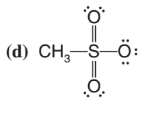# Problem: Give the formal charge (if one exists) on each atom of the following:

###### Problem Details

Give the formal charge (if one exists) on each atom of the following:What scientific concept do you need to know in order to solve this problem?

Our tutors have indicated that to solve this problem you will need to apply the Formal Charges concept. You can view video lessons to learn Formal Charges. Or if you need more Formal Charges practice, you can also practice Formal Charges practice problems.

What is the difficulty of this problem?

Our tutors rated the difficulty ofGive the formal charge (if one exists) on each atom of the f...as low difficulty.

How long does this problem take to solve?

Our expert Organic tutor, Jonathan took 1 minute and 1 second to solve this problem. You can follow their steps in the video explanation above.

What textbook is this problem found in?

Our data indicates that this problem or a close variation was asked in Organic Chemistry - Solomons 10th Edition. You can also practice Organic Chemistry - Solomons 10th Edition practice problems.